Elementary Algebra

# Chapter 10

Elementary AlgebraChapter 10

### Try It

10.1

$x = 9 , x = −9 x = 9 , x = −9$

10.2

$y = 11 , y = −11 y = 11 , y = −11$

10.3

$x = 5 2 , x = −5 2 x = 5 2 , x = −5 2$

10.4

$y = 3 3 , y = −3 3 y = 3 3 , y = −3 3$

10.5

$x = 7 , x = −7 x = 7 , x = −7$

10.6

$z = 6 , z = −6 z = 6 , z = −6$

10.7

no real solution

10.8

no real solution

10.9

$x = 2 10 , x = −2 10 x = 2 10 , x = −2 10$

10.10

$y = 2 7 , y = −2 7 y = 2 7 , y = −2 7$

10.11

$r = 6 5 5 , r = − 6 5 5 r = 6 5 5 , r = − 6 5 5$

10.12

$t = 8 3 3 , t = − 8 3 3 t = 8 3 3 , t = − 8 3 3$

10.13

$q = −6 , q = −4 q = −6 , q = −4$

10.14

$r = 8 , r = −2 r = 8 , r = −2$

10.15

$a = 3 + 3 2 , a = 3 − 3 2 a = 3 + 3 2 , a = 3 − 3 2$

10.16

$b = −2 + 2 10 , b = −2 − 2 10 b = −2 + 2 10 , b = −2 − 2 10$

10.17

$x = 1 3 + 5 3 , x = 1 3 − 5 3 x = 1 3 + 5 3 , x = 1 3 − 5 3$

10.18

$y = 3 4 + 7 4 , y = 3 4 − 7 4 y = 3 4 + 7 4 , y = 3 4 − 7 4$

10.19

$a = 5 + 2 5 , a = 5 − 2 5 a = 5 + 2 5 , a = 5 − 2 5$

10.20

$b = 3 + 4 2 , b = 3 − 4 2 b = 3 + 4 2 , b = 3 − 4 2$

10.21

no real solution

10.22

no real solution

10.23

$x = 3 + 2 3 , x = 3 − 2 3 x = 3 + 2 3 , x = 3 − 2 3$

10.24

$y = −6 + 4 2 , y = −6 − 4 2 y = −6 + 4 2 , y = −6 − 4 2$

10.25

$m = 7 , m = −3 m = 7 , m = −3$

10.26

$n = − 3 4 , n = − 7 4 n = − 3 4 , n = − 7 4$

10.27

$( y + 6 ) 2 ( y + 6 ) 2$

10.28

$( z + 4 ) 2 ( z + 4 ) 2$

10.29

$( a − 10 ) 2 ( a − 10 ) 2$

10.30

$( b − 2 ) 2 ( b − 2 ) 2$

10.31

$( m − 5 2 ) 2 ( m − 5 2 ) 2$

10.32

$( n + 13 2 ) 2 ( n + 13 2 ) 2$

10.33

$( p + 1 8 ) 2 ( p + 1 8 ) 2$

10.34

$( q − 1 3 ) 2 ( q − 1 3 ) 2$

10.35

$c = −5 , c = 1 c = −5 , c = 1$

10.36

$d = −9 , d = −1 d = −9 , d = −1$

10.37

$r = −2 , r = 6 r = −2 , r = 6$

10.38

$t = −1 , t = 11 t = −1 , t = 11$

10.39

no real solution

10.40

no real solution

10.41

$x = 8 ± 4 3 x = 8 ± 4 3$

10.42

$y = −4 ± 3 3 y = −4 ± 3 3$

10.43

$a = −7 , a = 3 a = −7 , a = 3$

10.44

$b = −10 , b = −2 b = −10 , b = −2$

10.45

$p = 5 2 ± 61 2 p = 5 2 ± 61 2$

10.46

$q = 7 2 ± 37 2 q = 7 2 ± 37 2$

10.47

$c = −3 ± 4 2 c = −3 ± 4 2$

10.48

$d = −7 , d = 11 d = −7 , d = 11$

10.49

$m = −4 ± 2 5 m = −4 ± 2 5$

10.50

$n = −2 , 8 n = −2 , 8$

10.51

$r = − 7 3 , r = 3 r = − 7 3 , r = 3$

10.52

$t = − 5 2 , t = 2 t = − 5 2 , t = 2$

10.53

$x = − 3 8 ± 201 8 x = − 3 8 ± 201 8$

10.54

$y = − 3 10 ± 209 10 y = − 3 10 ± 209 10$

10.55

$y = 2 3 , y = 1 y = 2 3 , y = 1$

10.56

$z = − 3 2 , z = 1 z = − 3 2 , z = 1$

10.57

$a = −3 , a = 5 a = −3 , a = 5$

10.58

$b = −6 , b = −4 b = −6 , b = −4$

10.59

$p = −4 ± 6 2 p = −4 ± 6 2$

10.60

$q = 11 ± 61 10 q = 11 ± 61 10$

10.61

$m = −6 ± 15 3 m = −6 ± 15 3$

10.62

$n = −2 ± 2 6 5 n = −2 ± 2 6 5$

10.63

no real solution

10.64

no real solution

10.65

$x = −1 ± 6 x = −1 ± 6$

10.66

$y = − 2 3 , y = 1 y = − 2 3 , y = 1$

10.67

$c = 2 ± 7 3 c = 2 ± 7 3$

10.68

$d = 2 3 , d = 0 d = 2 3 , d = 0$

10.69

$r = −5 r = −5$

10.70

$t = 4 5 t = 4 5$

10.71

no real solutions 2 1 no real solutions

10.72

2 no real solutions 1 2

10.73

factor Square Root Property Quadratic Formula

10.74

Quadratic Formula factoring Square Root Property

10.75

Two consecutive odd numbers whose product is 99 are 9 and 11, and $−9−9$ and $−11−11$.

10.76

Two consecutive even numbers whose product is 168 are 12 and 14,and $−12−12$ and $−14−14$.

10.77

The height of the triangle is 8 inches and the width is 52 inches.

10.78

The height of the triangle is 20 feet and the width is 11 feet.

10.79

The length of the shadow is 6.3 feet and the length of the flag pole is 18.9 ft.

10.80

The distance to the opposite corner is 3.2.

10.81

The width of the garden is 11 feet and the length is 18 feet.

10.82

The width of the tablecloth is 6.8 feet and the length is 11.8 feet.

10.83

The arrow will reach 180 on its way up in 3 seconds, and on the way down in 3.8 seconds.

10.84

The ball will reach 48 feet on its way up in .6 seconds and on the way down in 5.5 seconds.

10.85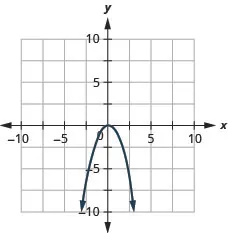10.86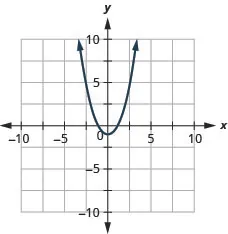10.87

up down

10.88

down up

10.89

$x=2x=2$ $(2,−7)(2,−7)$

10.90

$x=1x=1$ $(1,−5)(1,−5)$

10.91

$y : ( 0 , −8 ) ; x : ( −4 , 0 ) , ( 2 , 0 ) y : ( 0 , −8 ) ; x : ( −4 , 0 ) , ( 2 , 0 )$

10.92

$y : ( 0 , −12 ) ; x : ( 6 , 0 ) , ( −2 , 0 ) y : ( 0 , −12 ) ; x : ( 6 , 0 ) , ( −2 , 0 )$

10.93

$y : ( 0 , 4 ) ; x : none y : ( 0 , 4 ) ; x : none$

10.94

$y : ( 0 , −5 ) ; x : ( 5 , 0 ) ( −1 , 0 ) y : ( 0 , −5 ) ; x : ( 5 , 0 ) ( −1 , 0 )$

10.95

$y : ( 0 , −36 ) ; x : ( −6 , 0 ) y : ( 0 , −36 ) ; x : ( −6 , 0 )$

10.96

$y : ( 0 , 4 ) ; x : ( − 2 3 , 0 ) y : ( 0 , 4 ) ; x : ( − 2 3 , 0 )$

10.97

$y:(0,−8)y:(0,−8)$; $x:(2,0),(−4,0)x:(2,0),(−4,0)$;
axis: $x=−1x=−1$; vertex: $(−1,−9)(−1,−9)$;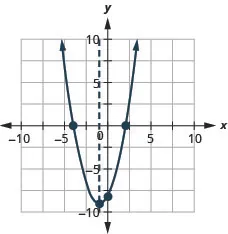10.98

$y:(0,12);x:(2,0),(6,0);y:(0,12);x:(2,0),(6,0);$
axis: $x=4;vertex:(4,−4)x=4;vertex:(4,−4)$;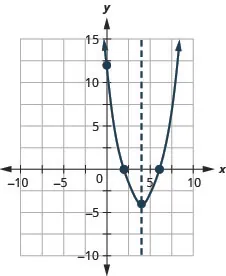10.99

$y:(0,−12);x:(2,0);y:(0,−12);x:(2,0);$
axis: $x=2;vertex:(2,0)x=2;vertex:(2,0)$;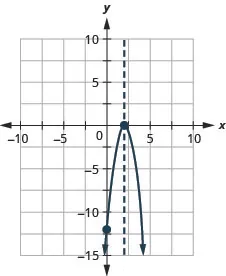10.100

$y:(0,1);x:(−15,0);y:(0,1);x:(−15,0);$
axis: $x=−15;vertex:(−15,0)x=−15;vertex:(−15,0)$;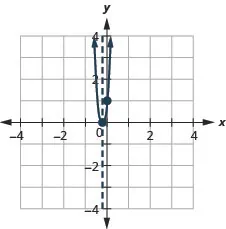10.101

$y:(0,5);x:none;y:(0,5);x:none;$
axis: $x=32;vertex:(32,12)x=32;vertex:(32,12)$;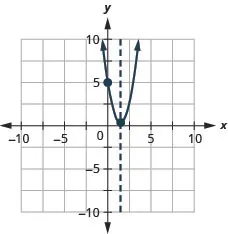10.102

$y:(0,−1);x:none;y:(0,−1);x:none;$
axis: $x=0;vertex:(0,−1)x=0;vertex:(0,−1)$;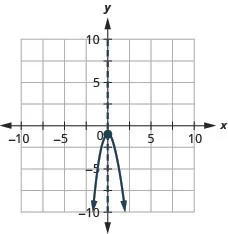10.103

$y:(0,3);x:(−1.6,0),(−0.4,0);y:(0,3);x:(−1.6,0),(−0.4,0);$
axis: $x=−1;vertex:(−1,−2)x=−1;vertex:(−1,−2)$;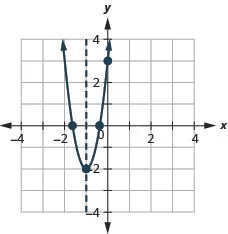10.104

$y:(0,5);x:(0.6,0),(−2.6,0);y:(0,5);x:(0.6,0),(−2.6,0);$
axis: $x=−1;vertex:(−1,8)x=−1;vertex:(−1,8)$;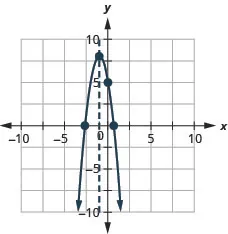10.105

The minimum value is $−4−4$ when $x=4x=4$.

10.106

The maximum value is 5 when $x=2x=2$.

10.107

It will take 4 seconds to reach the maximum height of 288 feet.

10.108

It will take 6.5 seconds to reach the maximum height of 676 feet.

### Section 10.1 Exercises

1.

$a = ± 7 a = ± 7$

3.

$r = ± 2 6 r = ± 2 6$

5.

$u = ± 10 3 u = ± 10 3$

7.

$m = ± 3 m = ± 3$

9.

no real solution

11.

$a = ± 2 5 a = ± 2 5$

13.

$p = ± 4 7 7 p = ± 4 7 7$

15.

$x = 1 , x = −5 x = 1 , x = −5$

17.

$u = 14 , u = −2 u = 14 , u = −2$

19.

$m = 6 ± 2 5 m = 6 ± 2 5$

21.

$r = 1 2 ± 3 2 r = 1 2 ± 3 2$

23.

$a = 7 ± 5 2 a = 7 ± 5 2$

25.

no real solution

27.

$m = 2 ± 2 2 m = 2 ± 2 2$

29.

$x = − 3 5 , x = 9 5 x = − 3 5 , x = 9 5$

31.

$r = ± 4 r = ± 4$

33.

$a = 4 ± 2 7 a = 4 ± 2 7$

35.

$w = 1 , w = 5 3 w = 1 , w = 5 3$

37.

$a = ± 3 2 a = ± 3 2$

39.

$p = 1 3 ± 7 3 p = 1 3 ± 7 3$

41.

no real solution

43.

$u = 7 ± 6 2 u = 7 ± 6 2$

45.

$m = 4 ± 2 3 m = 4 ± 2 3$

47.

$x = −3 , x = −7 x = −3 , x = −7$

49.

$c = ± 5 6 6 c = ± 5 6 6$

51.

no real solution

53.

4 feet

55.

### Section 10.2 Exercises

57.

$( a + 5 ) 2 ( a + 5 ) 2$

59.

$( m + 9 ) 2 ( m + 9 ) 2$

61.

$( m − 12 ) 2 ( m − 12 ) 2$

63.

$( p − 11 ) 2 ( p − 11 ) 2$

65.

$( x − 9 2 ) 2 ( x − 9 2 ) 2$

67.

$( p − 1 6 ) 2 ( p − 1 6 ) 2$

69.

$v = −10 , v = 4 v = −10 , v = 4$

71.

$u = −3 , u = 1 u = −3 , u = 1$

73.

$c = −1 , c = 13 c = −1 , c = 13$

75.

$x = −1 , x = 21 x = −1 , x = 21$

77.

no real solution

79.

no real solution

81.

$a = 5 ± 2 5 a = 5 ± 2 5$

83.

$u = 1 , u = 13 u = 1 , u = 13$

85.

$v = 9 2 ± 89 2 v = 9 2 ± 89 2$

87.

$x = −7 , x = 3 x = −7 , x = 3$

89.

$m = −11 , m = 1 m = −11 , m = 1$

91.

$c = −2 , c = 3 2 c = −2 , c = 3 2$

93.

$p = − 7 4 ± 161 4 p = − 7 4 ± 161 4$

95.

16 feet, 20 feet

97.

$−5−5$ $−5−5$ Answers will vary.

### Section 10.3 Exercises

99.

$m = −1 , m = 3 4 m = −1 , m = 3 4$

101.

$p = 1 2 , p = 3 p = 1 2 , p = 3$

103.

$p = −4 , p = −3 p = −4 , p = −3$

105.

$r = −3 , r = 11 r = −3 , r = 11$

107.

$u = −7 ± 73 6 u = −7 ± 73 6$

109.

$a = 3 ± 3 2 a = 3 ± 3 2$

111.

no real solution

113.

$v = −5 ± 65 2 v = −5 ± 65 2$

115.

$m = −1 , m = 3 4 m = −1 , m = 3 4$

117.

$c = − 3 4 c = − 3 4$

119.

$m = − 7 5 , m = 1 m = − 7 5 , m = 1$

121.

$p = −3 , p = 9 p = −3 , p = 9$

123.

$r = −3 ± 89 8 r = −3 ± 89 8$

125.

$a = −6 ± 26 2 a = −6 ± 26 2$

127.

$b = −2 ± 11 6 b = −2 ± 11 6$

129.

$x = −6 ± 42 4 x = −6 ± 42 4$

131.

no real solutions 1
2 no real solutions

133.

1 no real solutions
1 2

135.

factor square root

137.

factor square root
factor

139.

5 seconds, 8 seconds

141.

$−20,10−20,10$ $−20,10−20,10$

### Section 10.4 Exercises

143.

Two consecutive odd numbers whose product is 255 are 15 and 17, and $−15−15$ and $−17−17$.

145.

Two consecutive even numbers whose product is 624 are 24 and 26, and $−26−26$ and $−24−24$.

147.

Two consecutive odd numbers whose product is 483 are 21 and 23, and $−21−21$ and $−23−23$.

149.

The width of the triangle is 5 inches and the height is 18 inches.

151.

The leg of the right triangle is 1.7 feet and the hypotenuse is 3.4 feet.

153.

The length of the fence is 7.1 units.

155.

The width of the driveway is 10 feet and its length is 35 feet.

157.

The rocket will reach 1,200 feet on its way up in 2 seconds and on the way down in 38 seconds.

159.

70 seconds

161.

### Section 10.5 Exercises

163.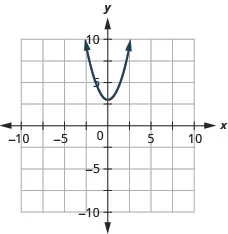165.

down

167.

up

169.

$x=−4x=−4$ $(−4,−17)(−4,−17)$

171.

$x=1x=1$ $(1,6)(1,6)$

173.

$y : ( 0 , 6 ) ; x : ( −1 , 0 ) , ( −6 , 0 ) y : ( 0 , 6 ) ; x : ( −1 , 0 ) , ( −6 , 0 )$

175.

$y : ( 0 , 19 ) ; x : none y : ( 0 , 19 ) ; x : none$

177.

$y : ( 0 , 25 ) ; x : ( 5 2 , 0 ) y : ( 0 , 25 ) ; x : ( 5 2 , 0 )$

179.

$y:(0,5);x:(−1,0),(−5,0);y:(0,5);x:(−1,0),(−5,0);$
axis: $x=−3;vertex:(−3,−4)x=−3;vertex:(−3,−4)$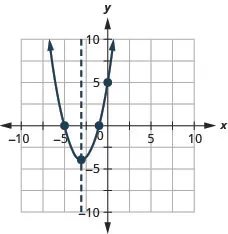181.

$y:(0,3);x:(−1,0),(−3,0);y:(0,3);x:(−1,0),(−3,0);$
axis: $x=−2;vertex:(−2,−1)x=−2;vertex:(−2,−1)$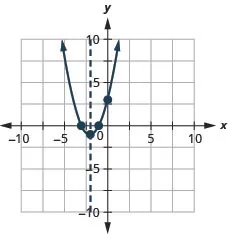183.

$y:(0,4)x:(−23,0);y:(0,4)x:(−23,0);$
axis: $x=−23;vertex:(−23,0)x=−23;vertex:(−23,0)$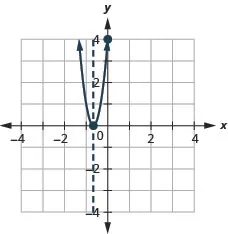185.

$y:(0,−7);x:none;y:(0,−7);x:none;$
axis: $x=1;vertex:(1,−6)x=1;vertex:(1,−6)$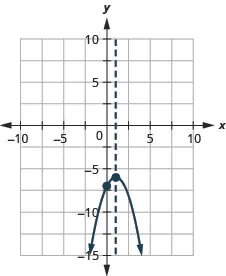187.

$y:(0,1);x:(1.7,0),(0.3,0);y:(0,1);x:(1.7,0),(0.3,0);$
axis: $x=1;vertex:(1,−1)x=1;vertex:(1,−1)$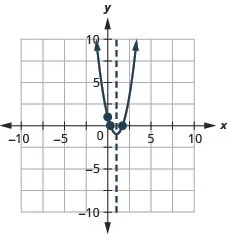189.

$y:(0,2)x:(1,0);y:(0,2)x:(1,0);$
axis: $x=1;vertex:(1,0)x=1;vertex:(1,0)$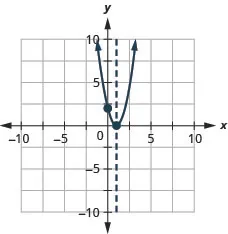191.

$y:(0,2)x:(−4.4,0),(0.4,0);y:(0,2)x:(−4.4,0),(0.4,0);$
axis: $x=−2;vertex:(−2,6)x=−2;vertex:(−2,6)$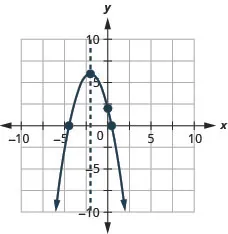193.

$y:(0,8);x:none;y:(0,8);x:none;$
axis: $x=1;vertex:(1,3)x=1;vertex:(1,3)$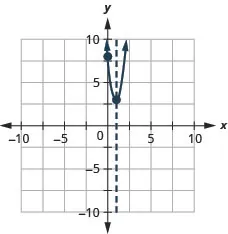195.

$y:(0,20)x:(−4.5,0),(−1.5,0);y:(0,20)x:(−4.5,0),(−1.5,0);$
axis: $x=−3;vertex:(−3,−7)x=−3;vertex:(−3,−7)$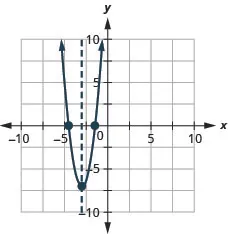197.

The minimum value is $−98−98$ when $x=−14x=−14$.

199.

The minimum value is 6 when $x=3x=3$.

201.

The maximum value is 16 when $x=0x=0$.

203.

In 5.3 sec the arrow will reach maximum height of 486 ft.

205.

20 computers will give the maximum of \$400 in receipts.

207.

The length of the side along the river of the corral is 120 feet and the maximum area is 7,200 sq ft.

209.

1.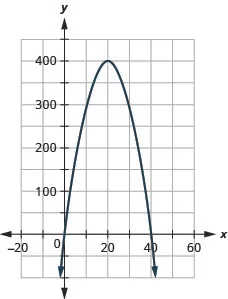2. $(0,0),(40,0)(0,0),(40,0)$
211.

### Review Exercises

213.

$x = ± 10 x = ± 10$

215.

$m = ± 2 10 m = ± 2 10$

217.

$a = ± 5 a = ± 5$

219.

no solution

221.

$v = ± 3 2 v = ± 3 2$

223.

$c = ± 4 5 5 c = ± 4 5 5$

225.

$p = 1 , 9 p = 1 , 9$

227.

$u = −1 ± 3 5 u = −1 ± 3 5$

229.

$x = 1 4 ± 3 4 x = 1 4 ± 3 4$

231.

$m = 7 ± 2 6 m = 7 ± 2 6$

233.

no solution

235.

$m = 3 ± 4 3 m = 3 ± 4 3$

237.

$a = − 3 2 , 3 4 a = − 3 2 , 3 4$

239.

$( x + 11 ) 2 ( x + 11 ) 2$

241.

$( m − 4 ) 2 ( m − 4 ) 2$

243.

$( a − 3 2 ) 2 ( a − 3 2 ) 2$

245.

$( p + 2 5 ) 2 ( p + 2 5 ) 2$

247.

$c = 1 , −21 c = 1 , −21$

249.

$x = −4 , 8 x = −4 , 8$

251.

no solution

253.

$v = 7 ± 3 2 v = 7 ± 3 2$

255.

$m = −9 , −1 m = −9 , −1$

257.

$a = 3 2 ± 41 2 a = 3 2 ± 41 2$

259.

$u = −6 ± 2 2 u = −6 ± 2 2$

261.

$p = 0 , 6 p = 0 , 6$

263.

$y = − 1 2 , 2 y = − 1 2 , 2$

265.

$c = − 1 3 ± 2 7 3 c = − 1 3 ± 2 7 3$

267.

$x = 1 4 , 1 x = 1 4 , 1$

269.

$r = −6 , 7 r = −6 , 7$

271.

$v = − 5 4 , 1 v = − 5 4 , 1$

273.

$m = −4 ± 10 3 m = −4 ± 10 3$

275.

no real solution

277.

$u = 5 ± 22 u = 5 ± 22$

279.

$p = 4 ± 6 5 p = 4 ± 6 5$

281.

$c = − 1 2 c = − 1 2$

283.

1 2 2 none

285.

287.

Two consecutive odd numbers whose product is 323 are 17 and 19, and $−17−17$ and $−19.−19.$

289.

The height of the banner is 13 cm and the length of the side is 54 cm.

291.

The lengths of the sides of the mosaic are 2.2 and 4.4 feet.

293.

The width of the front walk is 8.1 feet and its length is 30.8 feet.

295.

The ball will reach 384 feet on its way up in 4 seconds and on the way down in 6 seconds.

297.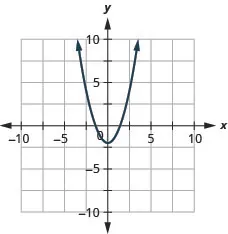299.

down

301.

up

303.

$x=3x=3$ $(3,17)(3,17)$

305.

$y : ( 0 , 5 ) ; x : ( 5 , 0 ) , ( −1 , 0 ) y : ( 0 , 5 ) ; x : ( 5 , 0 ) , ( −1 , 0 )$

307.

$y : ( 0 , 10 ) ; x : none y : ( 0 , 10 ) ; x : none$

309.

$y : ( 0 , 1 ) ; x : ( 1 4 , 0 ) y : ( 0 , 1 ) ; x : ( 1 4 , 0 )$

311.

$y:(0,15);x:(−3,0),(−5,0);y:(0,15);x:(−3,0),(−5,0);$
axis: $x=−4;vertex:(−4,−1)x=−4;vertex:(−4,−1)$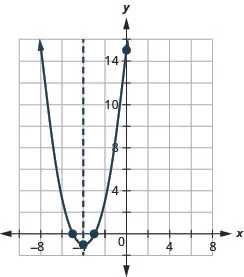313.

$y:(0,−16);x:(4,0);y:(0,−16);x:(4,0);$
axis: $x=4;vertex:(4,0)x=4;vertex:(4,0)$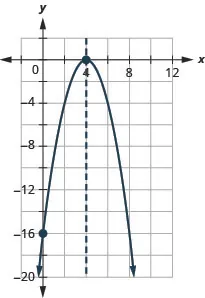315.

$y:(0,13);x:none;y:(0,13);x:none;$
axis: $x=−3;vertex:(−3,4)x=−3;vertex:(−3,4)$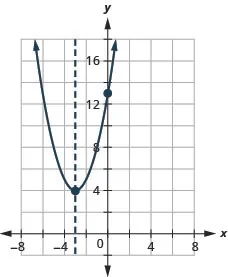317.

$y:(0,−11)x:(3.1,0),(0.9,0);y:(0,−11)x:(3.1,0),(0.9,0);$
axis: $x=2;vertex:(2,5)x=2;vertex:(2,5)$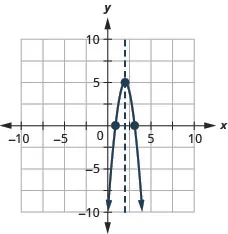319.

The minimum value is $−1−1$ when $x=−1x=−1$.

321.

In 3.5 seconds the ball is at its maximum height of 196 feet.

### Practice Test

323.

$w = −2 , −8 w = −2 , −8$

325.

$m = 1 , 3 2 m = 1 , 3 2$

327.

$n = −4 ± 7 3 n = −4 ± 7 3$

329.

no real solution

331.

2

333.

Two consecutive even number are $−20−20$ and $−18−18$ and 18 and 20.

335.

up $x=−1x=−1$ $(−1,5)(−1,5)$ $y:(0,8);x:noney:(0,8);x:none$ minimum value of 5 when $x=−1x=−1$

337.

up $x=−5x=−5$ $(−5,−1)(−5,−1)$ $y;(0,24);x:(−6,0),(−4,0)y;(0,24);x:(−6,0),(−4,0)$ minimum value of $−5−5$ when $x=−1x=−1$

339.

down $x=−4x=−4$
$(−4,32)(−4,32)$ $y;(0,16);x:(−9.7,0),(1.7,0)y;(0,16);x:(−9.7,0),(1.7,0)$
maximum value of $3232$ when $x=−4x=−4$

341.

$y:(0,9);x:(−34,0);y:(0,9);x:(−34,0);$
axis: $x=−34;vertex:(−34,0)x=−34;vertex:(−34,0)$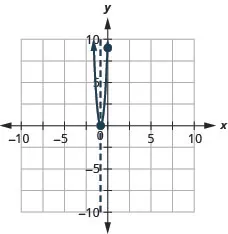Order a print copy

As an Amazon Associate we earn from qualifying purchases.